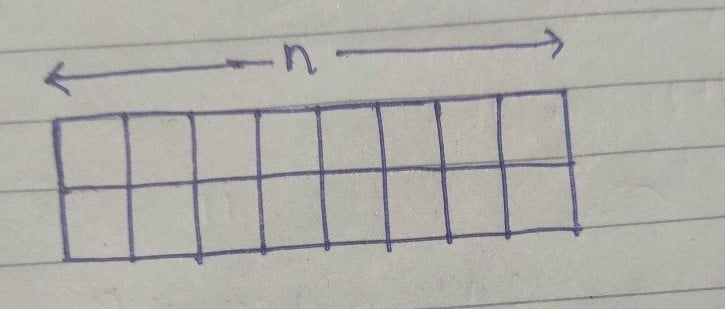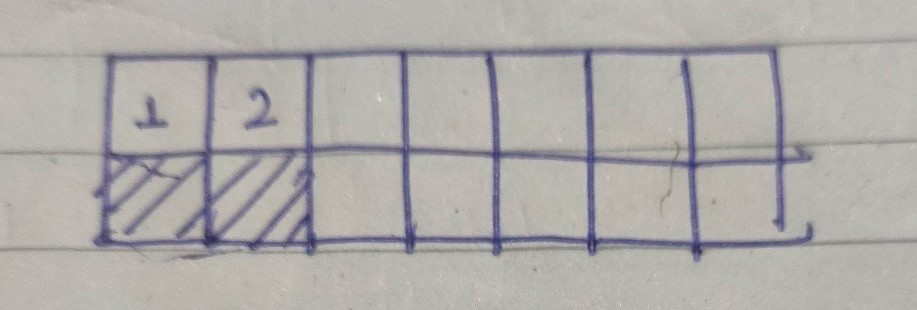TILING PROBLEM

We have a 2*n grid to be tiled.We have unlimited rectangular tiles of dimensions 1*2. Each tile can be placed either horizontally or vertically. Our aim is to fill the 2*n grid.

How many ways can we tile the 2*n grid using these tiles?

Example :

Input n = 4
Output: 5
Explanation:
For a 2 x 4 board, there are 5 ways
1) All 4 vertical
2) All 4 horizontal
3) First 2 horizontal, remaining 2 vertical
4) First 2 vertical, remaining 2 horizontal
5) Corner 2 vertical, middle 2 horizontal

Approach/Thinking Process :

1. We have only two choices : either place a horizontal tile or a vertical tile.

2. Suppose I place a vertical tile. Then we are left with 2*(n-1) grid to be filled.

3. Now, suppose if I place a horizontal tile. Then we are left with the following type of grid :If we will look carefully, 1 and 2 squares are not filled yet. To fill them we have to use only type of tiles which are given to us. Clearly, we can only fit those two squares by horizontal 1*2 tile only.

So, if we place that horizontal tile there, we are left with 2*(n-2) grid to be filled.

4. Now all the two cases possible are analyzed. It is obvious that for getting total number of arrangements possible we have to add the total number of arrangements of the two cases discussed above.

Recursive expression :

T(n) = T(n-1) + T(n-2) , where T(i) is number of arrangements possible when we have to fill 2*i grid.

Example of T(6):Observe that T(4) is computed twice, T(3) is computed 3 times, ….

We can modify the recursive procedure to store values that are already computed and avoid recomputation.

Efficient DP Solution :

• Base Case : When n=1 then we have 2*1 grid which can only be filled by vertical tile. So, T(1) = 1.

Also when n=2, then we have 2*2 grid which can be filled in two ways, either by placing two horizontal tiles or by placing two vertical tiles.

• Code :

Practice Problems :

Happy Coding 😊

By Programmers Army

Contributed by Shubham Kumar